GATE  >  Geology And Geophysics - (GG) 2018 GATE Paper (Practice Test)

# Geology And Geophysics - (GG) 2018 GATE Paper (Practice Test) - GATE

Test Description

## 95 Questions MCQ Test GATE Past Year Papers for Practice (All Branches) - Geology And Geophysics - (GG) 2018 GATE Paper (Practice Test)

Geology And Geophysics - (GG) 2018 GATE Paper (Practice Test) for GATE 2023 is part of GATE Past Year Papers for Practice (All Branches) preparation. The Geology And Geophysics - (GG) 2018 GATE Paper (Practice Test) questions and answers have been prepared according to the GATE exam syllabus.The Geology And Geophysics - (GG) 2018 GATE Paper (Practice Test) MCQs are made for GATE 2023 Exam. Find important definitions, questions, notes, meanings, examples, exercises, MCQs and online tests for Geology And Geophysics - (GG) 2018 GATE Paper (Practice Test) below.
Solutions of Geology And Geophysics - (GG) 2018 GATE Paper (Practice Test) questions in English are available as part of our GATE Past Year Papers for Practice (All Branches) for GATE & Geology And Geophysics - (GG) 2018 GATE Paper (Practice Test) solutions in Hindi for GATE Past Year Papers for Practice (All Branches) course. Download more important topics, notes, lectures and mock test series for GATE Exam by signing up for free. Attempt Geology And Geophysics - (GG) 2018 GATE Paper (Practice Test) | 95 questions in 180 minutes | Mock test for GATE preparation | Free important questions MCQ to study GATE Past Year Papers for Practice (All Branches) for GATE Exam | Download free PDF with solutions
 1 Crore+ students have signed up on EduRev. Have you?
Geology And Geophysics - (GG) 2018 GATE Paper (Practice Test) - Question 1

### “When she fell down the _______, she received many _______ but little help.” The words that best fill the blanks in the above sentence are

Geology And Geophysics - (GG) 2018 GATE Paper (Practice Test) - Question 2

### “In spite of being warned repeatedly, he failed to correct his _________ behaviour.” The word that best fills the blank in the above sentence is

Geology And Geophysics - (GG) 2018 GATE Paper (Practice Test) - Question 3

### For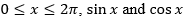are both decreasing functions in the interval ________.

Geology And Geophysics - (GG) 2018 GATE Paper (Practice Test) - Question 4

The area of an equilateral triangle is √3. What is the perimeter of the triangle?

Geology And Geophysics - (GG) 2018 GATE Paper (Practice Test) - Question 5

Arrange the following three-dimensional objects in the descending order of their volumes:
(i) A cuboid with dimensions 10 cm, 8 cm and 6 cm
(ii) A cube of side 8 cm
(iii) A cylinder with base radius 7 cm and height 7 cm
(iv) A sphere of radius 7 cm

Geology And Geophysics - (GG) 2018 GATE Paper (Practice Test) - Question 6

An automobile travels from city A to city B and returns to city A by the same route. The speed of the vehicle during the onward and return journeys were constant at 60 km/h and 90 km/h, respectively. What is the average speed in km/h for the entire journey?

Geology And Geophysics - (GG) 2018 GATE Paper (Practice Test) - Question 7

A set of 4 parallel lines intersect with another set of 5 parallel lines. How many parallelograms are formed?

Geology And Geophysics - (GG) 2018 GATE Paper (Practice Test) - Question 8

To pass a test, a candidate needs to answer at least 2 out of 3 questions correctly. A total of 6,30,000 candidates appeared for the test. Question A was correctly answered by 3,30,000 candidates. Question B was answered correctly by 2,50,000 candidates. Question C was answered correctly by 2,60,000 candidates. Both questions A and B were answered correctly by 1,00,000 candidates. Both questions B and C were answered correctly by 90,000 candidates. Both questions A and C were answered correctly by 80,000 candidates. If the number of students answering all questions correctly is the same as the number answering none, how many candidates failed to clear the test?

Geology And Geophysics - (GG) 2018 GATE Paper (Practice Test) - Question 9

If �� 2 + �� − 1 = 0 what is the value of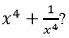Geology And Geophysics - (GG) 2018 GATE Paper (Practice Test) - Question 10

In a detailed study of annual crow births in India, it was found that there was relatively no growth during the period 2002 to 2004 and a sudden spike from 2004 to 2005. In another unrelated study, it was found that the revenue from cracker sales in India which remained fairly flat from 2002 to 2004, saw a sudden spike in 2005 before declining again in 2006. The solid line in the graph below refers to annual sale of crackers and the dashed line refers to the annual crow births in India. Choose the most appropriate inference from the above data.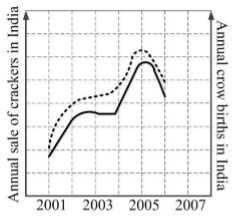Geology And Geophysics - (GG) 2018 GATE Paper (Practice Test) - Question 11

Which one of the following periods has the longest time duration?

Geology And Geophysics - (GG) 2018 GATE Paper (Practice Test) - Question 12

A siliciclastic sedimentary rock consisting predominantly of the same type of gravel-sized clasts is called

Geology And Geophysics - (GG) 2018 GATE Paper (Practice Test) - Question 13

Brown coal that has high moisture content and commonly retains many of the original wood fragments is called

Geology And Geophysics - (GG) 2018 GATE Paper (Practice Test) - Question 14

The speed of revolution of the Earth around the Sun is

Geology And Geophysics - (GG) 2018 GATE Paper (Practice Test) - Question 15

The geometrical factor for the following electrode configuration is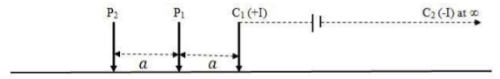Geology And Geophysics - (GG) 2018 GATE Paper (Practice Test) - Question 16

Which one of the following geophysical methods uses the physical property ‘Dielectric Constant’?

Geology And Geophysics - (GG) 2018 GATE Paper (Practice Test) - Question 17

Pascal second is a unit of

Geology And Geophysics - (GG) 2018 GATE Paper (Practice Test) - Question 18

Which one of the following statements is CORRECT?

Geology And Geophysics - (GG) 2018 GATE Paper (Practice Test) - Question 19

The geomorphic feature ‘horns’ are formed by

Geology And Geophysics - (GG) 2018 GATE Paper (Practice Test) - Question 20

A melanocratic porphyritic rock containing phenocrysts of biotite, with feldspar restricted to the groundmass, is called

Geology And Geophysics - (GG) 2018 GATE Paper (Practice Test) - Question 21

The supercontinent that existed in the late Mesoproterozoic to early Neoproterozoic time was

Geology And Geophysics - (GG) 2018 GATE Paper (Practice Test) - Question 22

The figure below shows the triple junction between three plates A, B and C. The boundary between the plates A and B is a ridge with a half-spreading rate of 4 cm/year. The A-C and B-C boundaries are collinear and orthogonal to the A-B ridge. The A-C boundary is a dextral transform fault with a relative velocity of 6 cm/year. The boundary between plates B and C is a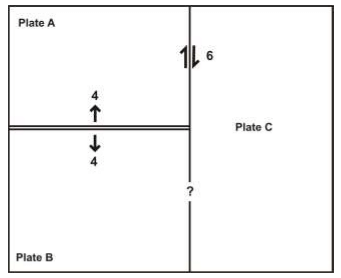Geology And Geophysics - (GG) 2018 GATE Paper (Practice Test) - Question 23

A rock follows Mohr-Coulomb failure criterion. Which one of the Mohr-Coulomb failure envelopes shown below allows failure of the rock under stress state Y, but not under stress state X?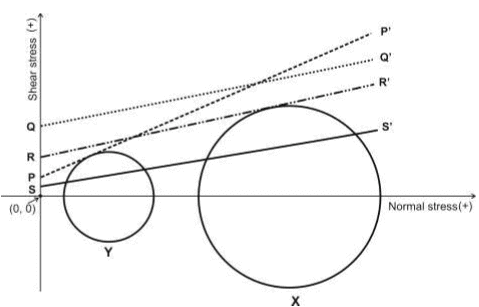Geology And Geophysics - (GG) 2018 GATE Paper (Practice Test) - Question 24

The maximum and the minimum principal stresses are denoted by σ1 and σ3, respectively. The differential stress can have an absolute value greater than σ1 when

Geology And Geophysics - (GG) 2018 GATE Paper (Practice Test) - Question 25

The geoid can be best defined as

Geology And Geophysics - (GG) 2018 GATE Paper (Practice Test) - Question 26

Gor a layered isotropic medium with a flat horizontal free surface, match the wave types listed in Group-I with their corresponding polarizations listed in Group II.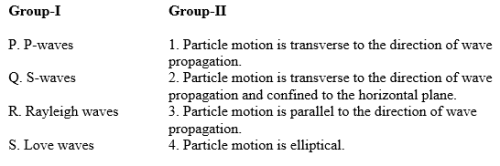Geology And Geophysics - (GG) 2018 GATE Paper (Practice Test) - Question 27

A ‘gentle’ fold with an interlimb angle equal to 160° appears tight (apparent interlimb angle equal to 20°) in horizontal section. According to the plunge of the fold axis, it can also be classified as

Geology And Geophysics - (GG) 2018 GATE Paper (Practice Test) - Question 28

The unit of shear modulus (rigidity modulus) is

Geology And Geophysics - (GG) 2018 GATE Paper (Practice Test) - Question 29

With increasing activity of silica, the CORRECT order of appearance of minerals in a weathering environment with constant ratio of activities of K+ and H+ is

Geology And Geophysics - (GG) 2018 GATE Paper (Practice Test) - Question 30

Match the items listed in Group-I with those listed in Group-II.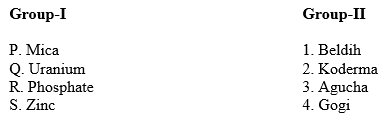Geology And Geophysics - (GG) 2018 GATE Paper (Practice Test) - Question 31

Which one of the following corrections is always added during reduction of the observed gravity data?

Geology And Geophysics - (GG) 2018 GATE Paper (Practice Test) - Question 32

The magnitudes of the total geomagnetic field at the equator and pole are denoted by BE and BP, respectively. Which one of the following is TRUE?

Geology And Geophysics - (GG) 2018 GATE Paper (Practice Test) - Question 33

Assume a flat earth with crustal thickness of 35 km and average crustal and upper mantle P-wave velocities of 6.4 km.s–1 and 8.1 km.s–1, respectively. The minimum distance from the epicenter of a near surface earthquake at which Pn-waves are observed is _______ km.

Geology And Geophysics - (GG) 2018 GATE Paper (Practice Test) - Question 34

Given that the velocity of P-waves in a sandstone matrix is 5600 m/s and that in oil is 1200 m/s, the velocity of P-wave propagation in oil saturated sandstone with 30% porosity is ___________ m/s. (Use Wyllie time average equation.)

Geology And Geophysics - (GG) 2018 GATE Paper (Practice Test) - Question 35

If the total porosity of a soil is 20%, its void ratio (%) is _________.

Geology And Geophysics - (GG) 2018 GATE Paper (Practice Test) - Question 36

Which one of the following Himalayan lithounits predates India-Eurasia collision?

Geology And Geophysics - (GG) 2018 GATE Paper (Practice Test) - Question 37

Which one of the following ore minerals shows internal reflection?

Geology And Geophysics - (GG) 2018 GATE Paper (Practice Test) - Question 38

Which one is CORRECT for the following equilibrium reaction between quartz and magnetite:

Si16O16O + Fe316O318O = Si16O18O + Fe316O4 ?

Geology And Geophysics - (GG) 2018 GATE Paper (Practice Test) - Question 39

Match the modes of life (listed in Group I) with the corresponding bivalve genera (listed in Group II).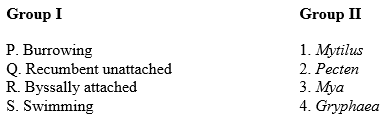Geology And Geophysics - (GG) 2018 GATE Paper (Practice Test) - Question 40

Based on the hypothetical litholog given below that shows a continuous succession of sedimentary rocks, which one of the following statements is CORRECT?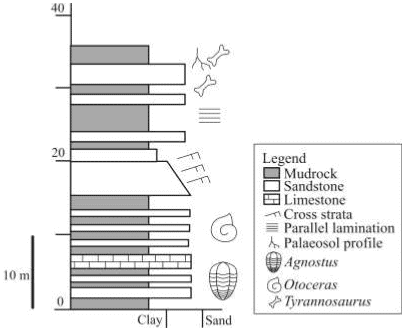Geology And Geophysics - (GG) 2018 GATE Paper (Practice Test) - Question 41

Which one of the following cladograms shows the CORRECT interrelationships among the major groups of vertebrates?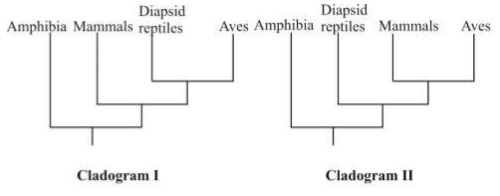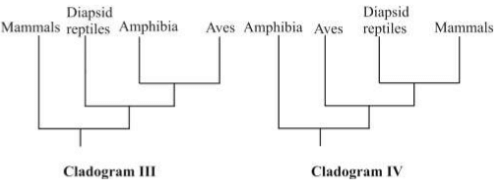Geology And Geophysics - (GG) 2018 GATE Paper (Practice Test) - Question 42

Which one of the following stratigraphic successions is in the CORRECT chronological order (from older to younger)?

Geology And Geophysics - (GG) 2018 GATE Paper (Practice Test) - Question 43

Match the items listed in Group I with their appropriate description listed in Group II.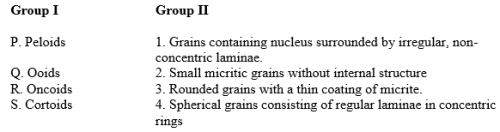Geology And Geophysics - (GG) 2018 GATE Paper (Practice Test) - Question 44

Which one of the following is an image rectification technique?

Geology And Geophysics - (GG) 2018 GATE Paper (Practice Test) - Question 45

Match the items listed in Group I with those in Group II.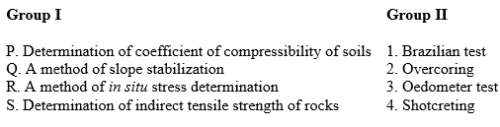Geology And Geophysics - (GG) 2018 GATE Paper (Practice Test) - Question 46

Match the items listed in Group I with those listed in Group II.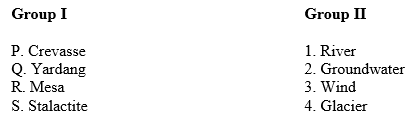Geology And Geophysics - (GG) 2018 GATE Paper (Practice Test) - Question 47

In the hypothetical isobaric ternary liquidus projection diagram given below, solid phases A, B, C, D and E exist in equilibrium with liquid. The reaction taking place at the isobaric invariant point W is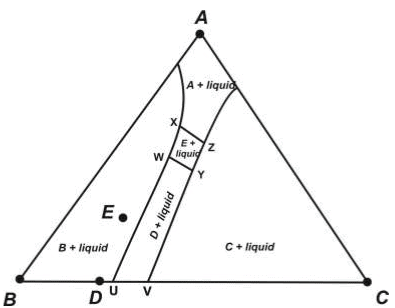Geology And Geophysics - (GG) 2018 GATE Paper (Practice Test) - Question 48

Match the optical properties listed in Group I with the corresponding mineral in Group II.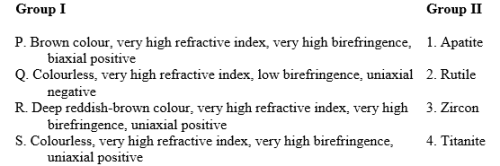Geology And Geophysics - (GG) 2018 GATE Paper (Practice Test) - Question 49

The reaction

muscovite + quartz = K-feldspar + sillimanite + water

Geology And Geophysics - (GG) 2018 GATE Paper (Practice Test) - Question 50

Match the items listed in Group I with those in Group II.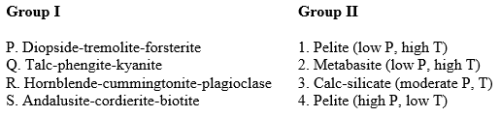Geology And Geophysics - (GG) 2018 GATE Paper (Practice Test) - Question 51

The figure below is a schematic section showing the initial stages of development of a thrust fault (FF’)  having a typical ramp and flat geometry, with the thrust sheet being transported from east to west. With respect to the synform and antiform created in Stage 2, which one of the options below is CORRECT for the next increment of movement on the fault plane?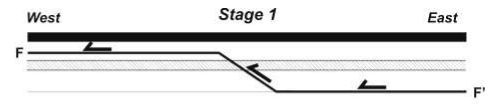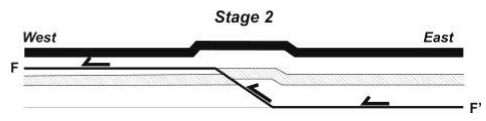Geology And Geophysics - (GG) 2018 GATE Paper (Practice Test) - Question 52

Which one of the following is the CORRECT chronological sequence for Iron formations?

Geology And Geophysics - (GG) 2018 GATE Paper (Practice Test) - Question 53

Assertion (a): High-temperature, low-pressure metamorphism occurs on the over-riding plate near convergent plate margins.
Reason (r): Partial melting in the mantle wedge generates magmas that rise to form the arc.

Geology And Geophysics - (GG) 2018 GATE Paper (Practice Test) - Question 54

Two coeval primary aqueous biphase fluid inclusions, X (liquid-rich) and Y (vapour-rich), occur in the same grain of the host mineral. Which one of the following situations most likely indicates boiling of the fluid?

Geology And Geophysics - (GG) 2018 GATE Paper (Practice Test) - Question 55

During bench blasting in a quarry, 50 kg of an explosive with a yield of 5 MegaJoule/kg is required to break 100 m3 of marble. In this case, the energy expended in breaking a unit volume of marble in MegaNewton/m2 would be _________________.

Geology And Geophysics - (GG) 2018 GATE Paper (Practice Test) - Question 56

The stretching lineation on the axial plane (S2) of a reclined fold on the S1 foliation makes an angle of 30° with the S1/S2 intersection lineation. The rake of the stretching lineation on the axial plane in degrees is ______________.

Geology And Geophysics - (GG) 2018 GATE Paper (Practice Test) - Question 57

A basaltic magma has an initial nickel concentration of 300 ppm. Olivine crystallizes from this magma by equilibrium crystallization (Case I) or fractional crystallization (Case II). Then, the absolute value of the difference between the nickel concentrations of the liquids remaining after 25% crystallization in these two cases is _______________. (Use KD,Ni olivine/melt = 10).

Geology And Geophysics - (GG) 2018 GATE Paper (Practice Test) - Question 58

The difference in the number of faces in forms {hkl} and {111} in the holosymmetric class of the isometric system is _______________.

Geology And Geophysics - (GG) 2018 GATE Paper (Practice Test) - Question 59

An inclined cylindrical confined aquifer has coefficient of permeability of 40 m/day. The horizontal distance between two vertical wells penetrating the aquifer is 800 m. The water surface elevations in the wells are 50 m and 45 m above a common horizontal datum. The absolute value of Darcy flux through the aquifer is ______________ m/day.

Geology And Geophysics - (GG) 2018 GATE Paper (Practice Test) - Question 60

The mass and volume of a natural soil sample are 2.1 kg and 1×10-3 m3, respectively. When fully dried, the mass of the soil sample becomes 2 kg without any change in volume. Assuming the specific gravity of soil particles to be 2.5, and water density of 1000 kg/m3, the degree of saturation of the natural soil sample is _______________%

Geology And Geophysics - (GG) 2018 GATE Paper (Practice Test) - Question 61

For a granitic rock mass, joint set number (Jn) = 9, joint water reduction factor (Jw) = 1, joint alteration number (Ja) = 1, stress reduction factor (SRF) = 1, rock quality designation (%) = 84 and joint roughness number (Jr) = 3. The Q-value as per Barton’s Q-system of rock mass classification (year 1974) is _______________.

Geology And Geophysics - (GG) 2018 GATE Paper (Practice Test) - Question 62

A sun synchronous satellite is at an altitude of 300 km and the spectrometer makes an angular coverage angle of 12°. The Swath (GFOV) of the satellite is ______________ km.

Geology And Geophysics - (GG) 2018 GATE Paper (Practice Test) - Question 63

The stability field boundary between two minerals A and B is linear with a positive slope in P-T space. The molar entropy of A and B are 85.5 and 92.5 Joules K-1, respectively and their respective molar volumes are 35.5 and 38.2 cc. The slope of the phase boundary in P-T space is _____________ bar K-1.

Geology And Geophysics - (GG) 2018 GATE Paper (Practice Test) - Question 64

Five moles of gas A (volume V1) and 3 moles of gas B (volume V2) were kept in separate containers. These two gases are completely transferred to a new container of volume V. Assuming isothermal condition, and that the work done is only mechanical, the entropy change of the system is ____________ Joules K-1. (R = 8.3 J K-1 mole-1)

Geology And Geophysics - (GG) 2018 GATE Paper (Practice Test) - Question 65

The value of Eh corresponding to the upper limit of natural surface aqueous environment at pH of 8.0 is _____________ V.

Geology And Geophysics - (GG) 2018 GATE Paper (Practice Test) - Question 66

The maximum number of linearly independent rows of an m × n matrix G where m > n is

Geology And Geophysics - (GG) 2018 GATE Paper (Practice Test) - Question 67

The impulse response of the Kirchhoff pre-stack time migration operator for non-zero offsets in a homogeneous and isotropic medium is _______.

Geology And Geophysics - (GG) 2018 GATE Paper (Practice Test) - Question 68

A solution to the eikonal equation |����| = 1⁄��0 for a homogeneous and isotropic medium in cartesian coordinates is

Geology And Geophysics - (GG) 2018 GATE Paper (Practice Test) - Question 69

The formula for the ‘forward’ Fourier transform is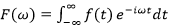and that for the ‘inverse’ Fourier transform is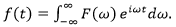Then, the forward Fourier transform of the function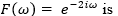is

Detailed Solution for Geology And Geophysics - (GG) 2018 GATE Paper (Practice Test) - Question 69

C (or) D

Geology And Geophysics - (GG) 2018 GATE Paper (Practice Test) - Question 70

Which one of the following rock types has the highest bulk magnetic susceptibility value?

Geology And Geophysics - (GG) 2018 GATE Paper (Practice Test) - Question 71

Figure 1 is a schematic diagram of four seismic events in t-x (time-offset) domain and Figure 2 is the result of transformation from t-x domain to f-kx (frequency-horizontal wavenumber) domain. Match the events in t-x domain in Figure 1 with their counterparts in f-kx domain in Figure 2.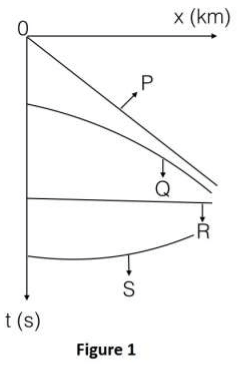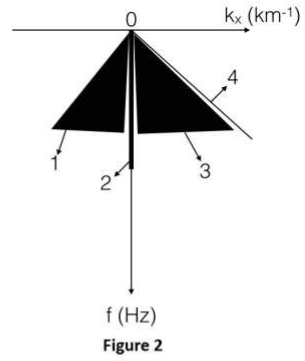Geology And Geophysics - (GG) 2018 GATE Paper (Practice Test) - Question 72

There is a change in the values of the bulk modulus and density across the Gutenberg discontinuity (from mantle to outer core). Which one of the following statements is CORRECT?

Geology And Geophysics - (GG) 2018 GATE Paper (Practice Test) - Question 73

Multi-electrode resistivity survey is carried out by placing 10 equispaced electrodes (denoted by arrows in the figure below) on the surface of the earth. The points of observations in the distance-apparent depth plane are marked as solid dots in the figure shown below. Considering the mid-point of the 4-electrode array as the point of observation in the lateral direction, identify the CORRECT electrode configuration used for the survey.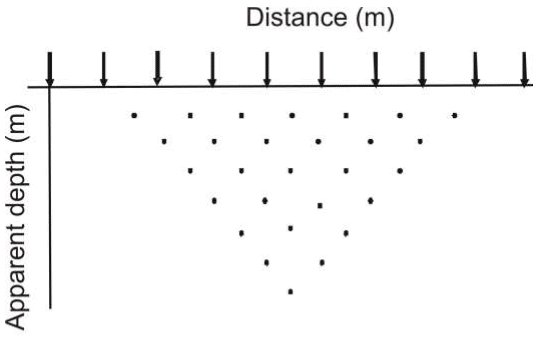Geology And Geophysics - (GG) 2018 GATE Paper (Practice Test) - Question 74

Match the Electromagnetic methods in Group I with the corresponding quantity measured by them given in Group IIGeology And Geophysics - (GG) 2018 GATE Paper (Practice Test) - Question 75

Dip angle electromagnetic response measured along a profile over multiple conductors is shown in the figure below. Which of the crossover points P, Q and R represent the CORRECT locations of conductors beneath them?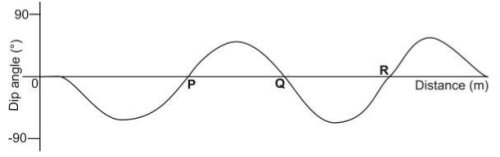Geology And Geophysics - (GG) 2018 GATE Paper (Practice Test) - Question 76

The effect of small scale near surface inhomogeneities can be removed from magnetic data by

Geology And Geophysics - (GG) 2018 GATE Paper (Practice Test) - Question 77

The frequencies of the primary magnetic field generated by worldwide thunderstorm activity vary in the range

Geology And Geophysics - (GG) 2018 GATE Paper (Practice Test) - Question 78

AssertionAssertion (a): The Static Self-Potential for a thick, clean freshwater bearing sandstone formation is positive.
Reason(r): Resistivity of the formation water is less than the resistivity of salt water mudfiltrate.

Geology And Geophysics - (GG) 2018 GATE Paper (Practice Test) - Question 79

Which one of the following well log responses characterizes an overpressured zone in the subsurface?

Geology And Geophysics - (GG) 2018 GATE Paper (Practice Test) - Question 80

The angle of inclination of the remanent magnetization measured on a basalt flow at a location P (28°N 85°E) is 40°. The palaeomagnetic latitude of the basalt flow is _______°N.

Geology And Geophysics - (GG) 2018 GATE Paper (Practice Test) - Question 81

Using the Gutenberg-Richter recurrence relationship, the mean annual rate of exceedance of earthquake occurrence in a seismic belt is 0.3 per year for an earthquake of magnitude 6.0. The return period for an earthquake of magnitude 6.0 in this belt is ______ years.

Geology And Geophysics - (GG) 2018 GATE Paper (Practice Test) - Question 82

In the figure below, Z denotes the depth to the center of a buried sphere from the surface and X1/2 denotes the half-width of the profile at half the maximum value of gravity. Then, the ratio Z/X1/2 is ________.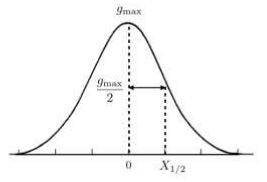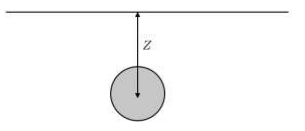Geology And Geophysics - (GG) 2018 GATE Paper (Practice Test) - Question 83

Two survey vessels with shipborne gravimeters are cruising towards each other at a speed of 6 knots each along an east-west course. The difference in gravity readings of the two gravimeters is 63.5 mGal at the point at which the survey vessels cross each other. The latitude along which the survey vessels are cruising is ______°N.

Geology And Geophysics - (GG) 2018 GATE Paper (Practice Test) - Question 84

A gravity reading is taken in a stationary helicopter hovering 1 km above mean-sea level at a particular location. The difference in the value of g measured in the helicopter and at mean sea level vertically beneath the helicopter is ____________ mGals.

Geology And Geophysics - (GG) 2018 GATE Paper (Practice Test) - Question 85

The P-wave velocity and the Poisson’s ratio for a homogeneous and isotropic sedimentary rock are 2500 m/s and 0.3, respectively. The S-wave velocity for the rock is ________m/s.

Geology And Geophysics - (GG) 2018 GATE Paper (Practice Test) - Question 86

A plane electromagnetic (EM) wave travelling vertically downwards with a frequency of 1000 Hz in a homogeneous medium has a skin depth of 100 m. The ratio of the amplitude of the EM wave at a depth of 75 m with respect to the amplitude at the Earth’s surface is

Geology And Geophysics - (GG) 2018 GATE Paper (Practice Test) - Question 87

A student interpreted a four layer Schlumberger resistivity sounding data and obtained the resistivities (r) and thicknesses (h) as follows: ρ1=100 Ωm, ρ2=20 Ωm, ρ3=1500 Ωm and ρ4=50 Ωm; h1=50 m, h2=10 m and h3=20 m. The same data is interpreted by another student who obtains ρ3=2000 Ωm. Then, according to the principle of equivalence, the value of h3 interpreted by the second student is ________m. (All other model parameters estimated by both the students are the same.)

Geology And Geophysics - (GG) 2018 GATE Paper (Practice Test) - Question 88

The apparent resistivities obtained at 0.1 Hz and 10 Hz in the frequency domain I.P. measurement are 100 Ωm and 80 Ωm, respectively. The Percentage Frequency Effect is _________.

Geology And Geophysics - (GG) 2018 GATE Paper (Practice Test) - Question 89

A 15 Volt power supply is applied across a cylindrical container (diameter = 0.20 m and length = 0.50 m). Currents of 750 mA and 500 mA are measured when the container is filled with (i) brine only, and (ii) rock sample fully saturated with brine, respectively. The formation factor of the rock sample is __________.

Geology And Geophysics - (GG) 2018 GATE Paper (Practice Test) - Question 90

The ratio of the number of daughter nuclides to the number of parent nuclides after a decay period of 3 half-lives is _______.

Geology And Geophysics - (GG) 2018 GATE Paper (Practice Test) - Question 91

Consider a laterally homogeneous and isotropic earth model with a flat horizontal surface and three horizontal layers underlain by a half-space. A seismic reflection survey was simulated on this model with the sources and receivers placed on the surface. The table below lists the root mean square (rms) velocities, Vrms, and zero-offset two-way traveltimes t0 for the three reflection events from the bottom of each of the three layers observed in a pre-stack CDP (CMP) gather. The interval velocity of the second layer is _______ m/s.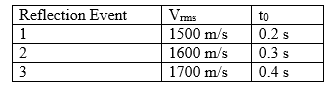Geology And Geophysics - (GG) 2018 GATE Paper (Practice Test) - Question 92

A spherically symmetric vector field ⃗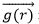is defined by the relationship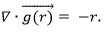The flux of the vector field through a sphere of unit radius is _________.

(Use �� = 3.14)

Geology And Geophysics - (GG) 2018 GATE Paper (Practice Test) - Question 93

A horizontally travelling surface wave with a wavelength of 20 m is attenuated by a linear and uniform receiver array consisting of 4 receivers if the minimum receiver spacing is ________ m.

Geology And Geophysics - (GG) 2018 GATE Paper (Practice Test) - Question 94

An end-on marine survey is carried out with equal and uniform shot and receiver spacing. If the total number of shots fired is 50 and a total of 10000 traces are recorded, the maximum fold for the survey is _______.

Geology And Geophysics - (GG) 2018 GATE Paper (Practice Test) - Question 95

The highest singular value of the matrix G =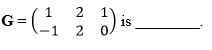## GATE Past Year Papers for Practice (All Branches)

407 docs|127 tests
Information about Geology And Geophysics - (GG) 2018 GATE Paper (Practice Test) Page
In this test you can find the Exam questions for Geology And Geophysics - (GG) 2018 GATE Paper (Practice Test) solved & explained in the simplest way possible. Besides giving Questions and answers for Geology And Geophysics - (GG) 2018 GATE Paper (Practice Test), EduRev gives you an ample number of Online tests for practice

## GATE Past Year Papers for Practice (All Branches)

407 docs|127 tests